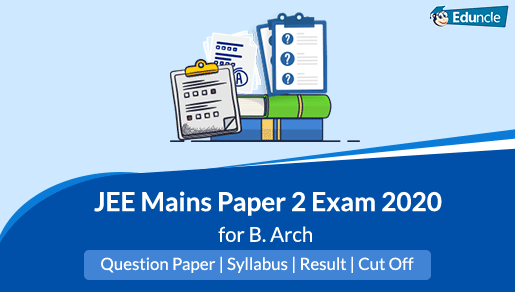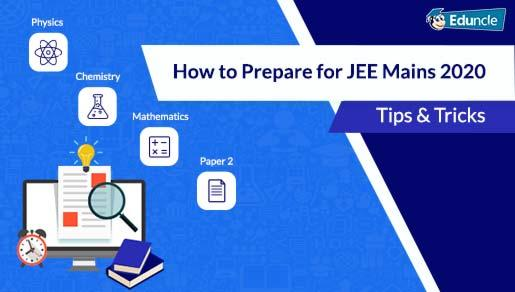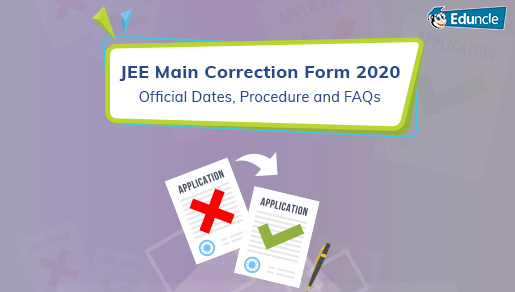Engineering Entrance (JEE) FollowEduncle posted an Article
January 9, 2020 • 3:42 pm

# IIT JEE Mathematics 2020 Important Topics, Preparation Tips & Tricks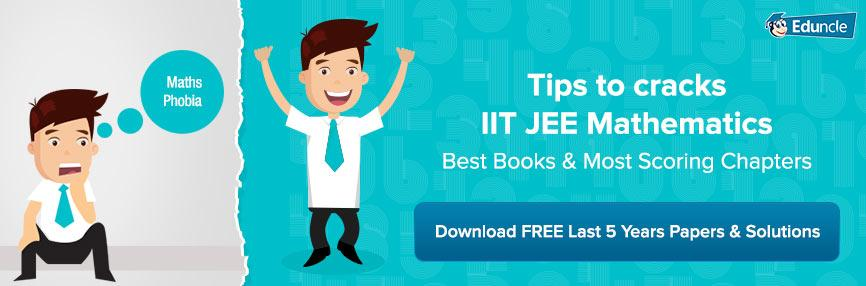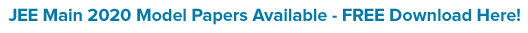IIT JEE is a high-level Engineering Entrance Exam to get admission into various IIT institutes. IITs are known for their standard and the quality education. It is the dream of every engineering aspirant to get the admission into IIT.

A proper strategy is essential to crack IIT Joint Entrance Exam, which is one of the toughest exams. One needs to clear the JEE Mains 2020 to become eligible for the JEE Advanced. IIT JEE Mathematics is the core subject to score well and crack the exam.

Many find Mathematics hard and can’t score enough to clear the minimum cutoff. To ease your preparation for JEE Mains and Advanced Maths, we are going to discuss IIT JEE Mathematics 2020 important topics and shortcut tricks for JEE Maths.

## IIT JEE Mathematics - How to Prepare for JEE Mains and Advanced?

Mathematics is the most scoring subject among all the three subjects asked in the Exam. You’ll need to have theoretical knowledge and problem-solving skills for the JEE Mathematics preparation.

It would be easier for you to prepare for the exam if you know the syllabus and exam pattern pretty well.

The very first thing you should do is to go through the  JEE Main Syllabus and JEE Advanced Syllabus. It will give you a clear understanding of what to study for JEE Exam preparation!

IIT JEE Mathematics syllabus can be divided into two parts-

IIT JEE Mains Mathematics

### Important Topics for IIT JEE Mains Mathematics

IIT JEE Mains Mathematics is a tricky part of the paper. The fear of Mathematics stops the candidates from attempting this section efficiently. However, candidates can score really well in Mathematics with little bit efforts and practice.

The JEE Mains Maths chapter wise weightage is mentioned below:

Chapter-1: Sets, Relations and Functions

This section carries 4% weightage for mains Exam.

Important topics in this section are Sets, Union, Intersection, and Complement of Sets and their Algebraic Properties, Power Set, Relation, Types of Relations, Equivalence Relations and Functions.

Chapter-2: Complex Numbers and Quadratic Equation

It is one of the important chapters which covers 5-6% weightage of the total marks.

Important topics are Representation, Algebra, Square Root of a Complex Number, Triangle Inequality, Quadratic Equations in Real and Complex Number System, Relation between Roots and Coefficients, Formation of Quadratic Equations with given roots.

Chapter-3: Matrices and Determinants

This section is given 6-7% weightage in mains syllabus and very important for the exam.

Algebra of Matrices & its types, Determinants, Adjoin and Evaluation of Square Matrix are important topics from this unit.

Chapter-4: Permutations and Combinations

This unit carries 3-4% weightage of the total marks.

Fundamental Principle, Permutation and Combination are important topics.

Chapter-5: Mathematical Induction

This section includes the principle of Mathematical Induction & its simple applications and carries 1-2% weightage.

Chapter-6: Binomial Theorem and Its Simple Applications

This unit carries 3-4% weightage in mains exam.

Binomial Theorem for a Positive Integral Index, Properties of Binomial Coefficients and its applications are some important topics from this unit.

Chapter-7: Sequences and Series

The JEE Mains weightage for this unit is 6-7%.

Arithmetic and Geometric Progressions, Relation between A.M. and G.M, Sum Up to “n” Terms of Special Series are important topics of this section.

Chapter-8: Limit, Continuity and Differentiation

It is an important chapter which carries 7-8% weightage

Important topics are Real-Valued Functions, Algebra of Functions, Polynomials, Rational, Trigonometric, Logarithmic and Exponential Functions, Inverse Functions, Graphs of Simple Functions, Limits, Continuity, Differentiation, Derivatives of order up to two, Rolle’s and Lagrange’s Mean Value Theorems.

Chapter-9: Integral Calculus

It is one of the most important chapters of the Mains syllabus, which has weightage 10-12% of the total marks.

Integral as an Anti-Derivative, Fundamental Integrals, Integration, Fundamental Theorem of Calculus, Properties of Definite Integrals, Evaluation of Definite Integrals are some important topics of this chapter.

Chapter-10: Differential Equations

This unit consists 6-7% weightage of the total marks.

Ordinary Differential Equations, Formation & Solution of Differential Equations are important topics in this unit.

Chapter-11: Co-Ordinate Geometry

This unit is very important which contains 10-12% weightage.

The important topics of this unit are Cartesian System, Straight Lines, Equations of Internal and External Bisectors of Angles between two lines, Coordinates of Centroid, Orthocenter, and Circumcenter of a Triangle, Circles, Conic Sections, Equations of Conic Sections (Parabola, Ellipse, and Hyperbola).

It is a highly scoring unit as it includes the application of the direct formulas. It requires an understanding of the Geometrical Shapes like Circle, Parabola, Triangle, Hyperbola etc.

Majority of questions asked from this topic are of the Straight Line and Circle.

Chapter-12: 3-D Geometry

This section carries 5-6% weightage.

Important topics from this section are Co-Ordinates of a Point in Space, Section Formula, Direction Ratios and Direction Cosines, The Angle between two Intersecting Lines, Skew Lines & its Equation, Equations of a Line and a Plane in different forms and Coplanar Lines.

Chapter-13: Vector Algebra

Vectors Algebra carries 3-4 % weightage in mains syllabus.

Vector and Scalars, Components of a Vector in 2D and 3D Space, Scalar and Vector Products are some must-read topics in this section.

Chapter-14: Statistics and Probability

This unit is given 4-5% weightage in syllabus.

Important topics for this section are Measures of Dispersion, Calculation of Standard Deviation, Variance and Mean Deviation, Probability, Baye’s Theorem, Bernoulli Trials and Binomial Distribution.

Chapter-15: Trigonometry

Trigonometry is an important section and carries 6-7% weightage in mains Exam.

This section consists important topics like Trigonometrical Identities, Equations, Functions and questions based on Heights & Distances.

Chapter-16: Mathematical Reasoning

The weightage of this section is 3-4%.

Statements, Logical Operations, Understanding of Tautology, Contradiction, Converse, and Contrapositive are some important topics of this section.

JEE Main 2020 Dates

Get Experts Designed Sample Papers for IIT JEE 2020 Exam. Download Free PDF with Detailed Solutions.

### Important Topics for IIT JEE Advanced Mathematics

JEE Advanced Mathematics consists following chapters:

Chapter-1: Algebra

Important topics are Complex Numbers, Quadratic Equations, Sequence & Series, Logarithms, Permutation & Combination, Binomial Theorem, Matrices & Determinants, Probability.

It is the chapter in which you can easily attain marks, all you need is swift calculation speed and clarity of the concepts.

Chapter-2: Trigonometry

Trigonometric Functions, Inverse Trigonometric Functions and Trigonometry Ratios are some of the most imperative areas of Trigonometry.

Chapter-3: Vectors

Properties of Vectors are important in this chapter.

Chapter-4: Differential Calculus

Important topics are Functions, Limits & Continuity, Derivatives.

The two-Section Differential and Integral deal with the study of changes and its application to solve the equation.

Chapter-5: Integral calculus

Integration, Application of Integration, Differential Equations are the must-read topics.

It is the most important unit for the JEE Mains and Advanced. It requires a clear fundamental theory of calculus.

Chapter-6: Analytical Geometry

Important topics are Two-Dimensional and Three-Dimensional Problems.

### Shortcut Tricks for IIT JEE Maths

It is very important to utilize every second in competitive exams like IIT JEE. It doesn’t matter, how you solved the answers, but the time taken to solve that problem is important.

In the Mathematics section, short tricks will help to solve question quickly and save time for the other section.

Have a look!

Learn all the important formulas and short tricks to save the time in the exam. Use Vedic Mathematics to improve the calculation speed and solve problems

For example, let us show you how to square two-digit numbers holding 5 at one’s place quickly?

When the last digit of two-digit number is 5 then It is sure that last two digits will be 25. Now, the remaining digit can be get by multiplying the second-place digit with its successor.

Example: Find the Square of 65. The last two digits of the answer will be 25. Remaining digits will be obtained by multiplying 6 with its successor which is 7 (6*7=42). The final answer will be square of 65 = 4225.

To solve Partial Differentiation, you should use Chain Rule.

L-Hospital Rules should be used to solve Limits Problem.

Make Algebra your weapon. Create the ability to picturize functions as graphs and learn to apply vertical and horizontal origin shifts carefully.

Integral Calculus can be scoring if you use tricks and some basic varieties of integral functions. You can save a lot of time by using properties and applying them wisely.

Solving the Co-ordinate Geometry questions would be fun if you understand the various forms of Conic Section and can convert the tougher ones to these basic forms. Co-ordinate Geometry questions can be solved easily using Complex Numbers.

To solve the Permutation-Combination and Probability problems, use basics of Bayes’ Theorem, Derangement and various ways of Distribution. You must be aware of cases, where objects are identical and not.

### IIT JEE Maths: Tricks to Score Best in Mains and Advanced

Although each chapter is equally covered in the exam but keep in mind the trend of last year’s pattern and prepare accordingly. Follow preparation tips for JEE Mathematics given below.

More importance should give to some topics like Algebra, Coordinate Geometry and 3-D Geometry & Vector.

You should focus more on the Calculus, Limit & Continuity, Sequence and Series which have more weightage in the syllabus.

Be well-aware of the result of the Algebraic Calculation and Properties of Conics for the Co-Ordinate Geometry Questions.

To score well in Trigonometry, learn all the important formulas and properties along with their graphs and substitution in this section.

Do not forget to review chapters like Mathematical Determinants, as these are relatively easy and can help you to score easily.

### What are the Best Books for JEE Mains and Advanced Mathematics?

Books play a vital role in the preparation for the exam. There are many books that you can refer to the IIT JEE Mathematics preparation.

For the preparation, important thing is that your basic concepts should be clear. Read the NCERT books for Class XI & XII and solve all the questions to improve the fundamentals.

You can check the -

Best Books for JEE Mains

### Revision Strategy for IIT JEE Mathematics [Bonus Tip]

For providing you a wise approach to crack IIT JEE Mathematics, Eduncle Experts have prepared the list of topics that you must do at first & at last. Check Out!

 S. No Must Do Topics Do Topics Last Do Topics 1 Algebra Limit & Continuity Permutations and Combinations 2 Integration Matrix & Determinants Quadratic Equation 3 Calculus (Differential & Integral) Analytical Geometry Vector 4 Complex Numbers Applications of Derivatives Mathematical Reasoning 5 Probability & Statistics Differentiations 3-D Geometry 6 Vector Algebra Sequence and Series Sets, Relations and Functions 7 Co-Ordinate Geometry _ Mathematical Induction 8 Trigonometry _ Binomial Theorem and Its Simple Applications

Here, we have discussed important topics, Short tricks and revision strategy and how to prepare for JEE Mains and Advanced mathematics. Hope It will help you in doing preparation for the JEE Mains as well as JEE Advanced Maths.

Do You Need Any Help in IIT JEE Preparations? Join Eduncle.com & Get Free Counselling by Experts.

Finally, one smart technique to prepare for the JEE Maths is to solve the previous year’s question papers. It will help you to know the question’s pattern asked in the previous year and will boost your score in the JEE Exam.

Keep Practicing and If you have any queries and suggestions, let us know in comment section below. If you like the article, please share it with your friends. Stay updated with Eduncle for the latest information.

You Might Also Like